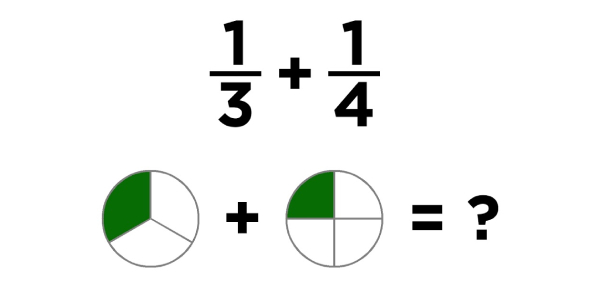# Adding And Subtracting Fractions Test! Quiz

10 Questions | Total Attempts: 169SettingsDo you comprehend how to add and subtract fractions? Could you pass this quiz? You can only add or subtract fractions if they have the same denominators. You must find the lowest common denominator before you add or subtract these fractions. Once the fractions have the same denominator, you can add or subtract as usual. If you want to put your knowledge to the test, this quiz can help.

• 1.
What is  4/5  +   1/2?
• A.

1 3/10

• B.

5/10

• C.

1 2/10

• D.

5/7

• 2.
7/9  --  2/3  =
• A.

1/3

• B.

1/9

• C.

5/6

• D.

5/9

• 3.
5/6   +   1/3  =
• A.

6/9

• B.

1

• C.

1 1/3

• D.

1 1/6

• 4.
2  1/2   --   1  5/10  =
• A.

1 1/10

• B.

1 3/5

• C.

1

• D.

1 3/10

• 5.
2  3/8   +   4  5/8  =
• A.

7

• B.

6 7/8

• C.

2 2/8

• D.

7 1/8

• 6.
4/10  +   8/10  =
• A.

1 1/10

• B.

1 1/5

• C.

1 2/20

• D.

1 2/5

• 7.
6/11   +    4/11   +    2/11  =
• A.

1 2/11

• B.

12/33

• C.

1

• D.

1 1/11

• 8.
5  2/3   --  3  1/2 =
• A.

2 1/2

• B.

2 1/3

• C.

2 1/6

• D.

2 1/5

• 9.
2/20  +    5/20   +  8/20  =
• A.

3/4

• B.

12/20

• C.

5/10

• D.

16/20

• 10.
When you add fractions with like denominators you always add the numerators and keep the denominators the same.  At the end of each problem, you need to write answers in the simplest form.
• A.

True

• B.

False

Related TopicsBack to top